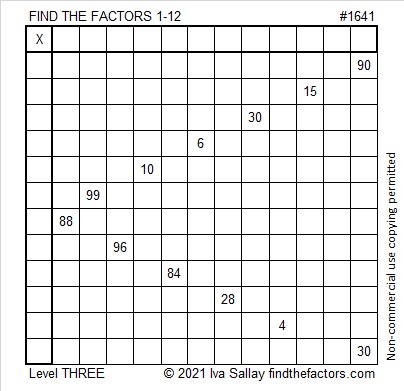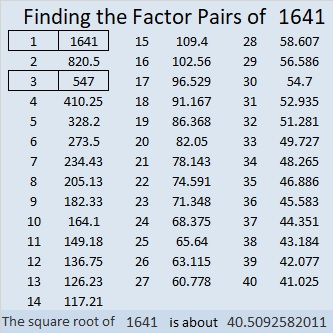# 1641 and Level 3

### Today’s Puzzle:

This is a level 3 puzzle so the clues have been placed so that you can know what order you should use the clues. Place the factors of 90 and 30 in the appropriate cells, then work your way down the puzzle cell by cell filling in the factors of the clues as you go.### Factors of 1641:

1 + 4 + 1 = 6, so 1641 is divisible by 3. (It isn’t necessary to include multiples of 3 in the sum to determine divisibility by 3.)

• 1641 is a composite number.
• Prime factorization: 1641 = 3 × 547.
• 1641 has no exponents greater than 1 in its prime factorization, so √1641 cannot be simplified.
• The exponents in the prime factorization are 1 and 1. Adding one to each exponent and multiplying we get (1 + 1)(1 + 1) = 2 × 2 = 4. Therefore 1641 has exactly 4 factors.
• The factors of 1641 are outlined with their factor pair partners in the graphic below.### More About the Number 1641:

1641 is the difference of two squares in two different ways:
821² – 820² = 1641, and
275² – 272² = 1641.

From OEIS.org we learn that the number formed from 1²6²4²1² is a perfect square:
Recall that 1² = 1; 6² = 36; 4² = 16; and 1² = 1. Those squares form the number, 136161.
Sure enough, 136161 = 369².